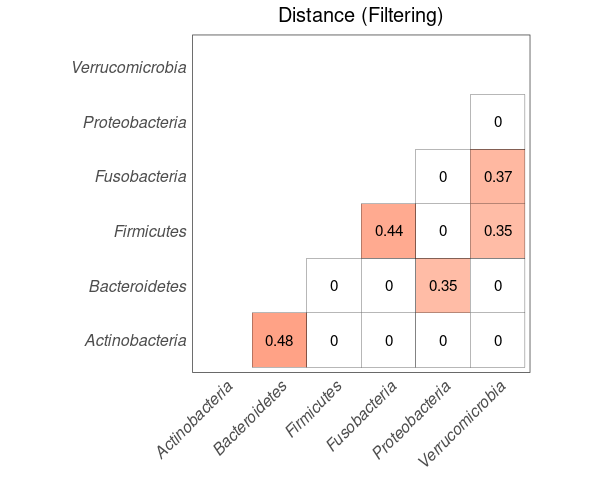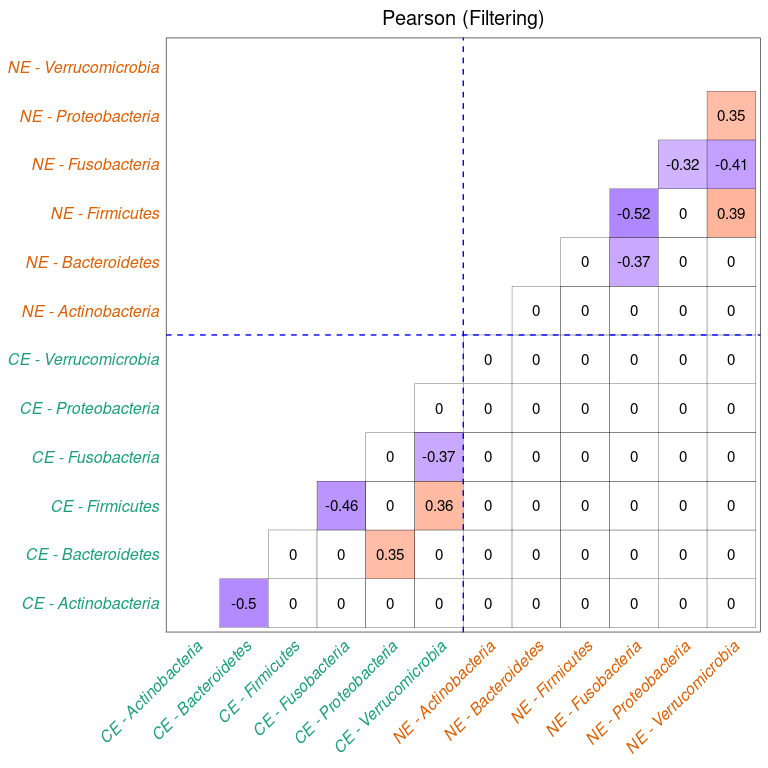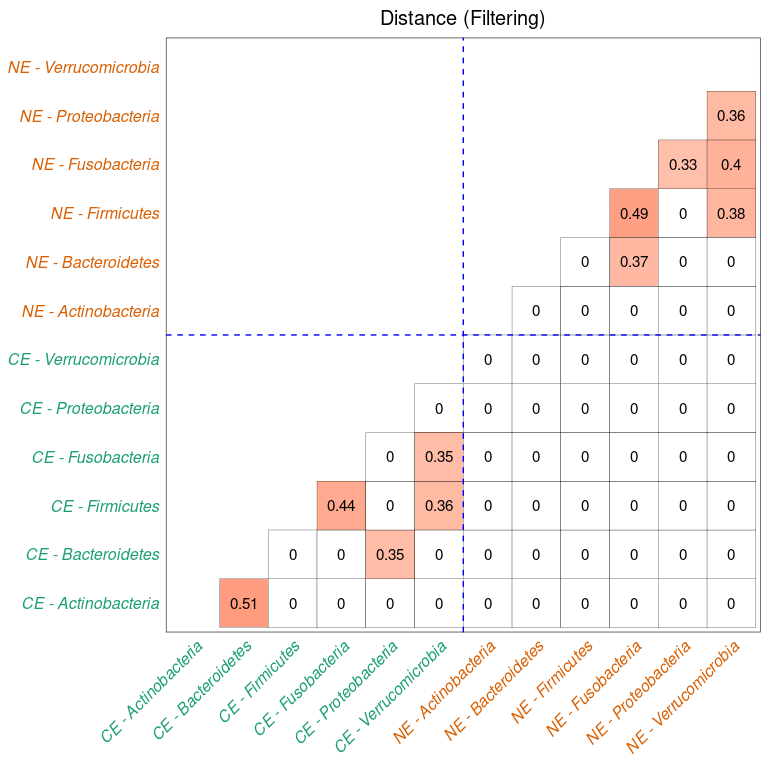# SECOM Tutorial

#### October 18, 2022

get_upper_tri = function(cormat){
cormat[lower.tri(cormat)] = NA
diag(cormat) = NA
return(cormat)
}

# 1. Introduction

Sparse Estimation of Correlations among Microbiomes (SECOM) (Lin, Eggesbø, and Peddada 2022) is a methodology that aims to detect both linear and nonlinear relationships between a pair of taxa within an ecosystem (e.g., gut) or across ecosystems (e.g., gut and tongue). SECOM corrects both sample-specific and taxon-specific biases and obtains a consistent estimator for the correlation matrix of microbial absolute abundances while maintaining the underlying true sparsity. For more details, please refer to the SECOM paper.

# 2. Installation

if (!requireNamespace("BiocManager", quietly = TRUE))
install.packages("BiocManager")
BiocManager::install("ANCOMBC")

library(ANCOMBC)

# 3. Example Data

The HITChip Atlas dataset contains genus-level microbiota profiling with HITChip for 1006 western adults with no reported health complications, reported in (Lahti et al. 2014). The dataset is also available via the microbiome R package (Lahti et al. 2017) in phyloseq (McMurdie and Holmes 2013) format.

data(atlas1006)

# Subset to baseline
tse = atlas1006[, atlas1006$time == 0] # Re-code the bmi group tse$bmi = recode(tse$bmi_group, obese = "obese", severeobese = "obese", morbidobese = "obese") # Subset to lean, overweight, and obese subjects tse = tse[, tse$bmi %in% c("lean", "overweight", "obese")]

# Create the region variable
tse$region = recode(as.character(tse$nationality),
Scandinavia = "NE", UKIE = "NE", SouthEurope = "SE",
CentralEurope = "CE", EasternEurope = "EE",
.missing = "unknown")

# Discard "EE" as it contains only 1 subject
# Discard subjects with missing values of region
cooccur_dist = res_dist$mat_cooccur # Filter by co-occurrence overlap = 10 corr_dist[cooccur_dist < overlap] = 0 df_dist = data.frame(get_upper_tri(corr_dist)) %>% rownames_to_column("var1") %>% pivot_longer(cols = -var1, names_to = "var2", values_to = "value") %>% filter(!is.na(value)) %>% mutate(value = round(value, 2)) tax_name = sort(union(df_dist$var1, df_dist$var2)) df_dist$var1 = factor(df_dist$var1, levels = tax_name) df_dist$var2 = factor(df_dist$var2, levels = tax_name) heat_dist_fl = df_dist %>% ggplot(aes(var2, var1, fill = value)) + geom_tile(color = "black") + scale_fill_gradient2(low = "blue", high = "red", mid = "white", na.value = "grey", midpoint = 0, limit = c(-1,1), space = "Lab", name = NULL) + scale_x_discrete(drop = FALSE) + scale_y_discrete(drop = FALSE) + geom_text(aes(var2, var1, label = value), color = "black", size = 4) + labs(x = NULL, y = NULL, title = "Distance (Filtering)") + theme_bw() + theme(axis.text.x = element_text(angle = 45, vjust = 1, size = 12, hjust = 1, face = "italic"), axis.text.y = element_text(size = 12, face = "italic"), strip.text.x = element_text(size = 14), strip.text.y = element_text(size = 14), legend.text = element_text(size = 12), plot.title = element_text(hjust = 0.5, size = 15), panel.grid.major = element_blank(), axis.ticks = element_blank(), legend.position = "none") + coord_fixed() heat_dist_fl# 5. Run SECOM on Multiple Ecosystems ## 5.1 Data manipulation To compute correlations whithin and across different ecosystems, one needs to make sure that there are samples in common across these ecosystems. # Select subjects from "CE" and "NE" tse1 = tse[, tse$region == "CE"]
cooccur_linear = res_linear$mat_cooccur # Filter by co-occurrence overlap = 10 corr_linear[cooccur_linear < overlap] = 0 df_linear = data.frame(get_upper_tri(corr_linear)) %>% rownames_to_column("var1") %>% pivot_longer(cols = -var1, names_to = "var2", values_to = "value") %>% filter(!is.na(value)) %>% mutate(var2 = gsub("\\...", " - ", var2), value = round(value, 2)) tax_name = sort(union(df_linear$var1, df_linear$var2)) df_linear$var1 = factor(df_linear$var1, levels = tax_name) df_linear$var2 = factor(df_linear$var2, levels = tax_name) txt_color = ifelse(grepl("CE", tax_name), "#1B9E77", "#D95F02") heat_linear_fl = df_linear %>% ggplot(aes(var2, var1, fill = value)) + geom_tile(color = "black") + scale_fill_gradient2(low = "blue", high = "red", mid = "white", na.value = "grey", midpoint = 0, limit = c(-1,1), space = "Lab", name = NULL) + scale_x_discrete(drop = FALSE) + scale_y_discrete(drop = FALSE) + geom_text(aes(var2, var1, label = value), color = "black", size = 4) + labs(x = NULL, y = NULL, title = "Pearson (Filtering)") + theme_bw() + geom_vline(xintercept = 6.5, color = "blue", linetype = "dashed") + geom_hline(yintercept = 6.5, color = "blue", linetype = "dashed") + theme(axis.text.x = element_text(angle = 45, vjust = 1, size = 12, hjust = 1, face = "italic", color = txt_color), axis.text.y = element_text(size = 12, face = "italic", color = txt_color), strip.text.x = element_text(size = 14), strip.text.y = element_text(size = 14), legend.text = element_text(size = 12), plot.title = element_text(hjust = 0.5, size = 15), panel.grid.major = element_blank(), axis.ticks = element_blank(), legend.position = "none") + coord_fixed() heat_linear_fl### Distance correlation with p-value filtering corr_dist = res_dist$dcorr_fl
cooccur_dist = res_dist$mat_cooccur # Filter by co-occurrence overlap = 10 corr_dist[cooccur_dist < overlap] = 0 df_dist = data.frame(get_upper_tri(corr_dist)) %>% rownames_to_column("var1") %>% pivot_longer(cols = -var1, names_to = "var2", values_to = "value") %>% filter(!is.na(value)) %>% mutate(var2 = gsub("\\...", " - ", var2), value = round(value, 2)) tax_name = sort(union(df_dist$var1, df_dist$var2)) df_dist$var1 = factor(df_dist$var1, levels = tax_name) df_dist$var2 = factor(df_dist\$var2, levels = tax_name)
txt_color = ifelse(grepl("CE", tax_name), "#1B9E77", "#D95F02")

heat_dist_fl = df_dist %>%
ggplot(aes(var2, var1, fill = value)) +
geom_tile(color = "black") +
scale_fill_gradient2(low = "blue", high = "red", mid = "white",
na.value = "grey", midpoint = 0, limit = c(-1,1),
space = "Lab", name = NULL) +
scale_x_discrete(drop = FALSE) +
scale_y_discrete(drop = FALSE) +
geom_text(aes(var2, var1, label = value), color = "black", size = 4) +
labs(x = NULL, y = NULL, title = "Distance (Filtering)") +
theme_bw() +
geom_vline(xintercept = 6.5, color = "blue", linetype = "dashed") +
geom_hline(yintercept = 6.5, color = "blue", linetype = "dashed") +
theme(axis.text.x = element_text(angle = 45, vjust = 1, size = 12, hjust = 1,
face = "italic", color = txt_color),
axis.text.y = element_text(size = 12, face = "italic",
color = txt_color),
strip.text.x = element_text(size = 14),
strip.text.y = element_text(size = 14),
legend.text = element_text(size = 12),
plot.title = element_text(hjust = 0.5, size = 15),
panel.grid.major = element_blank(),
axis.ticks = element_blank(),
legend.position = "none") +
coord_fixed()

heat_dist_fl# Session information

sessionInfo()
R version 4.2.1 (2022-06-23)
Platform: x86_64-pc-linux-gnu (64-bit)
Running under: Ubuntu 20.04.5 LTS

Matrix products: default
BLAS:   /home/biocbuild/bbs-3.15-bioc/R/lib/libRblas.so
LAPACK: /home/biocbuild/bbs-3.15-bioc/R/lib/libRlapack.so

locale:
 LC_CTYPE=en_US.UTF-8       LC_NUMERIC=C
 LC_TIME=en_GB              LC_COLLATE=C
 LC_MONETARY=en_US.UTF-8    LC_MESSAGES=en_US.UTF-8
 LC_PAPER=en_US.UTF-8       LC_NAME=C
 LC_MEASUREMENT=en_US.UTF-8 LC_IDENTIFICATION=C

attached base packages:
 stats4    stats     graphics  grDevices utils     datasets  methods
 base

other attached packages:
 caret_6.0-93                   lattice_0.20-45
 DT_0.25                        mia_1.4.0
 MultiAssayExperiment_1.22.0    TreeSummarizedExperiment_2.4.0
 Biostrings_2.64.1              XVector_0.36.0
 SingleCellExperiment_1.18.1    SummarizedExperiment_1.26.1
 Biobase_2.56.0                 GenomicRanges_1.48.0
 GenomeInfoDb_1.32.4            IRanges_2.30.1
 S4Vectors_0.34.0               BiocGenerics_0.42.0
 MatrixGenerics_1.8.1           matrixStats_0.62.0
 forcats_0.5.2                  stringr_1.4.1
 dplyr_1.0.10                   purrr_0.3.5
 tibble_3.1.8                   ggplot2_3.3.6
 tidyverse_1.3.2                ANCOMBC_1.6.4

loaded via a namespace (and not attached):
 estimability_1.4.1          ModelMetrics_1.2.2.2
 coda_0.19-4                 bit64_4.0.5
 knitr_1.40                  irlba_2.3.5.1
 multcomp_1.4-20             DelayedArray_0.22.0
 data.table_1.14.4           rpart_4.1.16
 hardhat_1.2.0               RCurl_1.98-1.9
 doParallel_1.0.17           generics_0.1.3
 ScaledMatrix_1.4.1          TH.data_1.1-1
 RSQLite_2.2.18              proxy_0.4-27
 future_1.28.0               bit_4.0.4
 tzdb_0.3.0                  xml2_1.3.3
 lubridate_1.8.0             assertthat_0.2.1
 DirichletMultinomial_1.38.0 viridis_0.6.2
 gargle_1.2.1                gower_1.0.0
 xfun_0.33                   hms_1.1.2
 jquerylib_0.1.4             evaluate_0.17
 fansi_1.0.3                 dbplyr_2.2.1
 Rmpfr_0.8-9                 CVXR_1.0-10
 ellipsis_0.3.2              crosstalk_1.2.0
 backports_1.4.1             energy_1.7-10
 permute_0.9-7               deldir_1.0-6
 sparseMatrixStats_1.8.0     vctrs_0.4.2
 cachem_1.0.6                withr_2.5.0
 checkmate_2.1.0             emmeans_1.8.1-1
 vegan_2.6-4                 treeio_1.20.2
 cluster_2.1.4               gsl_2.1-7.1
 ape_5.6-2                   lazyeval_0.2.2
 crayon_1.5.2                recipes_1.0.2
 pkgconfig_2.0.3             labeling_0.4.2
 nlme_3.1-160                vipor_0.4.5
 nnet_7.3-18                 rlang_1.0.6
 globals_0.16.1              lifecycle_1.0.3
 sandwich_3.0-2              modelr_0.1.9
 rsvd_1.0.5                  cellranger_1.1.0
 rngtools_1.5.2              Matrix_1.5-1
 boot_1.3-28                 zoo_1.8-11
 reprex_2.0.2                base64enc_0.1-3
 png_0.1-7                   viridisLite_0.4.1
 rootSolve_1.8.2.3           bitops_1.0-7
 pROC_1.18.0                 blob_1.2.3
 DelayedMatrixStats_1.18.2   doRNG_1.8.2
 decontam_1.16.0             parallelly_1.32.1
 jpeg_0.1-9                  DECIPHER_2.24.0
 beachmat_2.12.0             scales_1.2.1
 memoise_2.0.1               magrittr_2.0.3
 plyr_1.8.7                  zlibbioc_1.42.0
 compiler_4.2.1              RColorBrewer_1.1-3
 lme4_1.1-30                 cli_3.4.1
 lmerTest_3.1-3              listenv_0.8.0
 htmlTable_2.4.1             Formula_1.2-4
 MASS_7.3-58.1               mgcv_1.8-40
 tidyselect_1.2.0            stringi_1.7.8
 highr_0.9                   yaml_2.3.6
 BiocSingular_1.12.0         latticeExtra_0.6-30
 ggrepel_0.9.1               grid_4.2.1
 sass_0.4.2                  tools_4.2.1
 lmom_2.9                    future.apply_1.9.1
 parallel_4.2.1              rstudioapi_0.14
 foreach_1.5.2               foreign_0.8-83
 gridExtra_2.3               gld_2.6.5
 prodlim_2019.11.13          farver_2.1.1
 digest_0.6.30               lava_1.6.10
 Rcpp_1.0.9                  broom_1.0.1
 scuttle_1.6.3               httr_1.4.4
 Rdpack_2.4                  colorspace_2.0-3
 rvest_1.0.3                 fs_1.5.2
 splines_4.2.1               yulab.utils_0.0.5
 tidytree_0.4.1              expm_0.999-6
 scater_1.24.0               Exact_3.2
 xtable_1.8-4                gmp_0.6-6
 jsonlite_1.8.2              nloptr_2.0.3
 timeDate_4021.106           ipred_0.9-13
 R6_2.5.1                    Hmisc_4.7-1
 pillar_1.8.1                htmltools_0.5.3
 glue_1.6.2                  fastmap_1.1.0
 minqa_1.2.4                 BiocParallel_1.30.4
 BiocNeighbors_1.14.0        class_7.3-20
 codetools_0.2-18            mvtnorm_1.1-3
 utf8_1.2.2                  bslib_0.4.0
 numDeriv_2016.8-1.1         ggbeeswarm_0.6.0
 DescTools_0.99.46           interp_1.1-3
 survival_3.4-0              rmarkdown_2.17
 munsell_0.5.0               e1071_1.7-11
 GenomeInfoDbData_1.2.8      iterators_1.0.14
 haven_2.5.1                 reshape2_1.4.4
 gtable_0.3.1                rbibutils_2.2.9